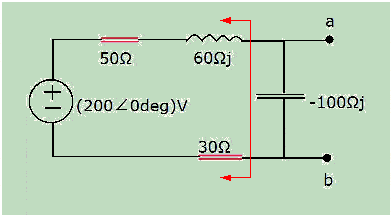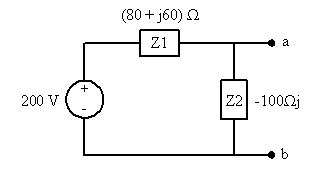# AC Circuit with Source Transformation; find Thevenin equivalent

• Engineering

## Homework Statement

Use source transformation to find the Thevenin equivalent circuit with respect to terminals, a, b.

[Broken]

## Homework Equations

Voltage Division: (V in)*(R1/R1+R2)

Thevenin / Norton / source transformation procedures

RTh = RNo

VTh = INo*RNo

Polar Form conversion:

Z = (R2 + X2)1/2

θ = arctan(j / R)

For Complex numbers:

j*j = -1

1/j = -j

## The Attempt at a Solution

I simplified the circuit to this first:

[Broken]

For source transformation, you find VTh by removing the load and then finding the drop from A back to the source right?

And you find RTh by finding the eq resistance from A to B with the voltage source suppressed right?

So trying to get VTh for the final answer, I think I do this:

[Broken]

because I'll want the drop from A right?

so for VTh:

(200∠0deg)V* [(50Ω+60Ωj)/(80Ω-40Ωj)]
in polar form is

(200∠0deg)V* [ (78.1∠50.1)/(61.4∠26.5) ]

= (254∠23.6deg)V

So I think the drop from A is

(200∠0)V - (254∠23.6deg)V

-> VTh = (-54 ∠ -23.6 deg)V

Now for RTh:

RTh = [ (1/80Ω + 60Ωj) + (-1/100Ωj) ]-1
so

= (100Ωj - 80Ω - 60Ω)/(8000Ωj2j + 6000Ω2j2)

=[ (40Ωj - 80Ω)/(8000Ω2j - 6000Ω2) ] * [ (-8000Ω2j - 6000Ω2)/-8000Ω2j - 6000Ω2) ]

= (-320000Ω3j2 - 240000Ω3j + 640000Ω3j + 480000Ω3) / (-64000000Ω4j2 + 36000000Ω4)

= [(80 x 1043 + (40 x 1043]/(100 x 106Ω4

RTh = 0.008Ω + 0.004Ωj

If I did anything wrong, I hope I am on the right track at least?? Thank you

Last edited by a moderator:

gneill
Mentor
The final results that you've obtained are not good.

Your first simplification is okay, but you can simplify it further by noting that you can form the Thevenin equivalent that combines the rest of the impedances except the capacitor into a single Thevenin impedance. In the figure below, that would be the Thevenin equivalent for everything left of the red arrow.Then you have a voltage divider situation:and finding the Thevenin equivalent of that should be straight forward.

#### Attachments

Wow, I sure missed that hard.

I still may need more help with source transformation itself.

To find the Thevenin Voltage now for this, you find the voltage across the top impedance (80Ω + 60Ωj) ?

So with voltage division that would be

= 200∠0*(80+60j/80-40j)

=> 200∠0*(100∠36.8 / 89.4∠-26.5)

= (223∠63.3)V ?

(Which looks strange since the magnitude of this is higher than that of the source)

gneill
Mentor
Wow, I sure missed that hard.

I still may need more help with source transformation itself.

To find the Thevenin Voltage now for this, you find the voltage across the top impedance (80Ω + 60Ωj) ?
Which impedance is a-b across?
So with voltage division that would be

= 200∠0*(80+60j/80-40j)

=> 200∠0*(100∠36.8 / 89.4∠-26.5)

= (223∠63.3)V ?

(Which looks strange since the magnitude of this is higher than that of the source)
While that's the wrong impedance to be taking the voltage across, it is not strange to find voltages in an RLC circuit that are higher than the source voltage. This is due to resonance effects (energy storage and swapping between the L and C components).

Okay, that is helpful to know, even though I did wrong impedance.

So then the Thevenin voltage is:

200∠0*(0 -100j/80-40j)

=> 200∠0*(100∠ -90 / 89.4 ∠ -26.5 )

= (2.23∠-63.5)V ?

By the way, I had for finding polar form of the -100j capacitor arctan(-100/0), can i just take limit instead so you avoid dividing by 0 (because there is no real resistance component)?

gneill
Mentor
Okay, that is helpful to know, even though I did wrong impedance.

So then the Thevenin voltage is:

200∠0*(0 -100j/80-40j)

=> 200∠0*(100∠ -90 / 89.4 ∠ -26.5 )

= (2.23∠-63.5)V ?
The angle looks good, but I think you've lost a factor of 100 on the magnitude.
By the way, I had for finding polar form of the -100j capacitor arctan(-100/0), can i just take limit instead so you avoid dividing by 0 (because there is no real resistance component)?
You could, but it's probably easier to simply recognize that any vector on the imaginary axis of the complex plane must have an angle of ±##\pi/2##.

So the Thevenin voltage is

= (223∠-63.5)V then.

This still looks really similar for the other impedance too though.

The Thevenin resistance (impedance) is still basically finding [ (1/80Ω + 60Ωj) + (-1/100Ωj) ]-1 also, right?

gneill
Mentor
So the Thevenin voltage is

= (223∠-63.5)V then.

This still looks really similar for the other impedance too though.
Yup. A coincidence due to choice of component values.
The Thevenin resistance (impedance) is still basically finding [ (1/80Ω + 60Ωj) + (-1/100Ωj) ]-1 also, right?

Yup.

I redid that for the Thevenin resistance now and got nearly the exact same answer as before, (except off by a factor of 10 for the final answer.)

I got

RTh = 0.08Ω + 0.04Ωj

gneill
Mentor
No, your Rth is not right. It should be much larger in magnitude.

Oh no, I forgot to divide the ^-1 power out so it was actually 1/(0.08Ω + 0.04Ωj) all along. My bad.

so

RTh = 12.5Ω + 25Ωj ?

gneill
Mentor
Oh no, I forgot to divide the ^-1 power out so it was actually 1/(0.08Ω + 0.04Ωj) all along. My bad.

so

RTh = 12.5Ω + 25Ωj ?
No, that's still to small in magnitude.

You have (80 + j60)Ω in parallel with -j100Ω. Show your work, one step at a time and annotate it with what you're doing for each step.

Okay. It seems I did make another mistake with forgetting to flip over the fraction from parallel before multiplying conjugate, so I did it again and will type out everything and all the zeroes here too to be sure:

RTh = [ (1/80Ω + 60Ωj) + (-1/100Ωj) ]-1

= (100Ωj - 80Ωj - 60Ωj)/(8000Ω2j + 6000Ω2j2) , adding fractions, and disregarding the whole thing to the power of -1 for now.

= (40Ωj - 80Ω)/(8000Ω2j - 6000Ω2) , simplifying the fraction, 100 - 60 on top, simplifying j*j for denominator,

Switching them around now because it was to the power -1 becomes

= (8000Ω2j - 6000Ω2)/(40Ωj - 80Ω)

Multiplying by opposite complex conjugate now means:

= (8000Ω2j - 6000Ω2)/(40Ωj - 80Ω) * (-40Ωj - 80Ω)/(-40Ωj - 80Ω)

= ( -320000Ω3j2 - 640000Ω3j + 240000Ω3j + 480000Ω3)/(-1600Ω2j2 - 3200Ω2j + 3200Ω2j + 6400Ω2)

Simplifying j*j = -1 means

= (320000Ω3j + 640000Ω3j + 240000Ω3j + 480000Ω3)/(1600Ω2 - 3200Ω2j + 3200Ω2j + 6400Ω2)

Now dividing out 100 from everything means

= (100/100) * (3200Ω3j + 6400Ω3j+ 2400Ω3j + 4800Ω3)/(16Ω2 - 32Ω2j + 32Ω2j + 64Ω2)

Combining like terms now means:

= (8000Ω3 + 8800Ω3j)/80Ω2

which reduces to

100Ω + 110Ωj

gneill
Mentor
Okay. It seems I did make another mistake with forgetting to flip over the fraction from parallel before multiplying conjugate, so I did it again and will type out everything and all the zeroes here too to be sure:

RTh = [ (1/80Ω + 60Ωj) + (-1/100Ωj) ]-1

= (100Ωj - 80Ωj - 60Ωj)/(8000Ω2j + 6000Ω2j2) , adding fractions, and disregarding the whole thing to the power of -1 for now.
The -80Ω term in the numerator should not be imaginary.
= (40Ωj - 80Ω)/(8000Ω2j - 6000Ω2) , simplifying the fraction, 100 - 60 on top, simplifying j*j for denominator,

Switching them around now because it was to the power -1 becomes

= (8000Ω2j - 6000Ω2)/(40Ωj - 80Ω)
Okay so far.
Multiplying by opposite complex conjugate now means:

= (8000Ω2j - 6000Ω2)/(40Ωj - 80Ω) * (-40Ωj - 80Ω)/(-40Ωj - 80Ω)

= ( -320000Ω3j2 - 640000Ω3j + 240000Ω3j + 480000Ω3)/(-1600Ω2j2 - 3200Ω2j + 3200Ω2j + 6400Ω2)

Simplifying j*j = -1 means

= (320000Ω3j + 640000Ω3j + 240000Ω3j + 480000Ω3)/(1600Ω2 - 3200Ω2j + 3200Ω2j + 6400Ω2)
There should be no "j" left on the 320000Ω3 term, since j*j = -1 as you stated above.

You should combine all the like terms at this point to simplify to a complex#/complex# form. You should get:
$$\frac{(800000 - 400000j)Ω^3}{8000Ω^2}$$
Then, since the denominator is purely real, divide the numerator terms accordingly.

I'm doing it on paper too (although in pen) and I still left out the j on the term when I added it, but I changed the sign on the 640000Ω3 for no reason there by mistake on the second line you quoted so it's really

(320000Ω3 - 640000Ω3j + 240000Ω3j + 480000Ω3)/(1600Ω2 - 3200Ω2j + 3200Ω2j + 6400Ω2)

Now it simplies to

(100/100)* (8000Ω3 - 4000Ω3j)/80Ω2

like you said

So RTh = 100Ω - 50Ωj

Thanks again for being patient with me.